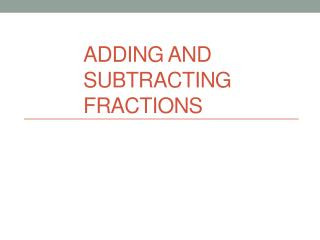DownloadDownload PresentationDownload Presentation- - - - - - - - - - - - - - - - - - - - - - - - - - - E N D - - - - - - - - - - - - - - - - - - - - - - - - - - -
##### Presentation Transcript

2. NS 2.1 Solve problems involving addition, subtraction, multiplication, and division of positive fractions and explain why a particular operation was used for a given situation. • Today’s objective: use our previous topic of equivalent fractions to help add and subtract fractions. • Learning target: Answer at least 3 of the 4 fraction addition/subtraction problems correctly on the exit ticket.

3. Let’s use pizzas cut up into slices • This pizza has been cut into 8 equal slices. A single slice is what fraction of the entire pizza? • Each slice is ________ of the entire pizza.

4. At a party, there are a pepperoni pizza and a mushroom pizza. Both are cut into 8 equal slices. I eat 3 slices of pepperoni and 1 slice of cheese. Write an equation to calculate the total fraction of a pizza that I ate. =

5. But what do we do when the one pizza is cut into a different amount of slices than another pizza? • Let’s say that again I eat 3 slices of pepperoni and 1 slice of cheese. Write a new equation to calculate the total fraction of a pizza that I ate. • What can we do to make it easier to compare the amount eaten from each pizza? • Cut the cheese pizza so it has the same number of slices as the pepperoni pizza.

6. Make the cheese pizza have 8 slices instead of 4: Now instead of , write it as When we cut the pizzas to have the same number of slices, the mathematical operation we did was finding a common denominator

7. If the fractions do not already have a common denominator, change them so that they do. • Add or subtract the numerators like normal but keep the denominator the same. • How do we add and subtract fractions?

8. Since you adding or subtracting pieces, the numerator changes. • However, since all pieces will be the same size, the denominator remains the same. 5 8

9. Direct Station • We will practice solving word problems which involve fractions.

10. Collaborative Station • Use the spinners to create fractions. For example, if you spin a 3 and then a 4, the fraction would be ¾ . • For each problem, create two fractions, then add them. • Write down the problem and the answer, including finding a common denominator if necessary.

11. Collaborative Station Example: • Partner A spins a 1 and a 2, creating ½ . • Partner B spins a 5 and a 3, creating • Both partners write down • They find the LCM is 6, so they rewrite their problem as • They solve their equation to get the answer

12. Independent Station • We are switching to ST Math’s unit on adding and subtracting fractions. • Write down your calculations on your paper.